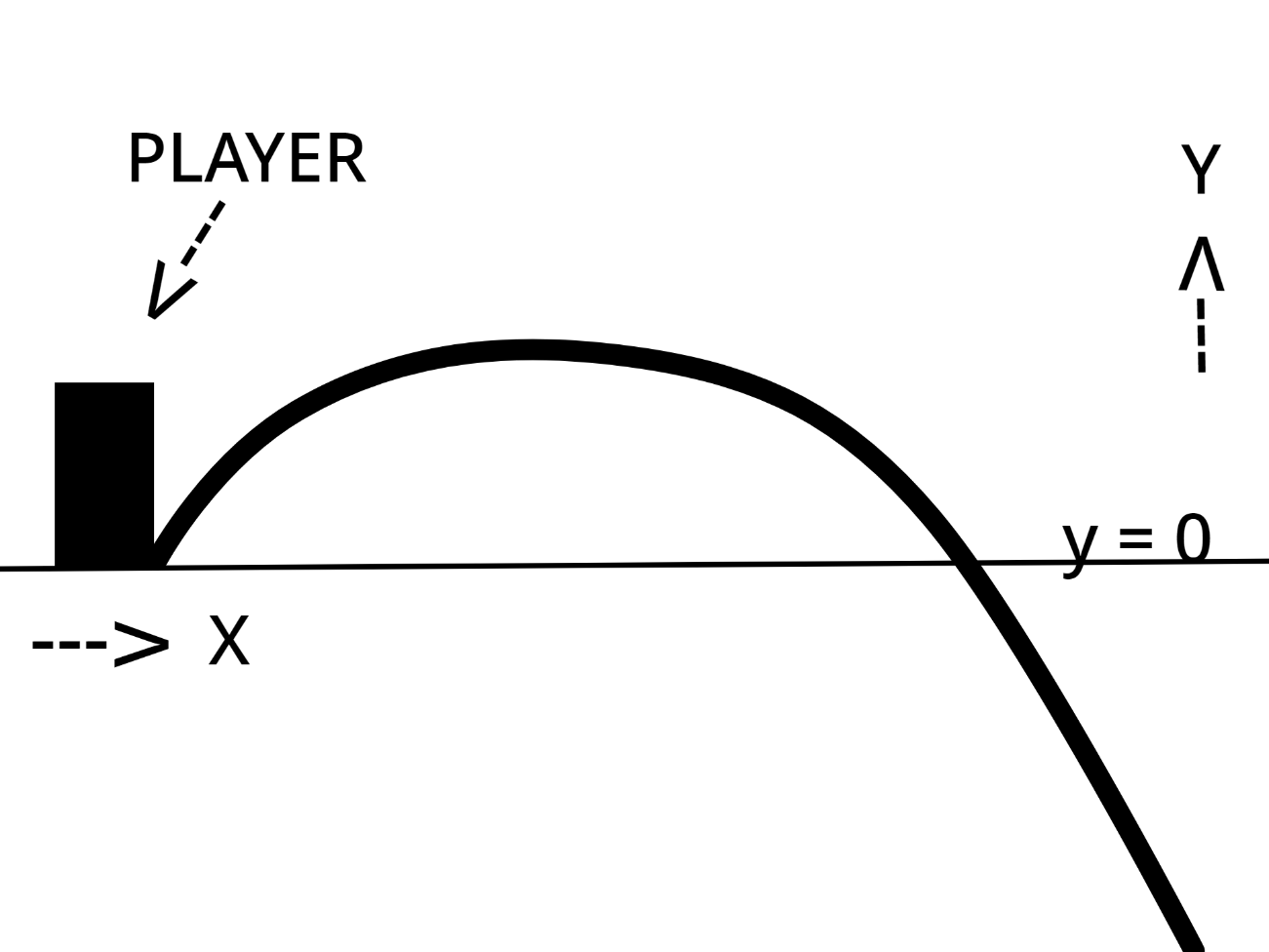# How can I get the height of a player after he traveled a N distance

Hi there! I would like to know how to get the height of a player after he traveled a N distance after jumping. This is hard to explain so please say to me if you don’t understand.

Note: the gravity, player walkspeed and player jump power are default. So the walkspeed is 15, the jump power is 50 and the gravity is 196,2.

Do you want the full trajectory or maybe the maximum height?

I would like to get the heigh of a player if he jump into the void and walk a distance (like 5 or 12 studs)

You can get the height of the player when he start to fall ?

I would like a fuction which return the heigh of a player n seconds after he jump into the void.

note: the player is at 0,0,0 before falling.

2 Likes

Try this :

``````Humanoid.StateChanged:Connect(function(OldState, NewState)
if NewState == Enum.HumanoidStateType.Freefall then
HeightOfThePlayer = Character:GetPrimaryPartCFrame().Position.Y
end
end)
``````

And you are french ?
If yes you can create a topic in the french categorie because im french too !

You did not understand. This is an estimation of if a player fall. No players have to fall to get the value of the function. It should return y value if I enter x value.1 Like

Yes okay you want the trajectory then I’d say.

Typically these curves are something like:

y(t) = y_0 + v_y * t - 1/2 g t²
x(t) = x_0 + v_x * t

Where (x_0, y_0) is the start position, (v_x, v_y) is the start velocity, g is acceleration due to gravity.

I think this is a good start but characters don’t seem to follow typical momentum conservation without extra body movers / constraints so you may need to account for that too.

2 Likes